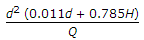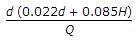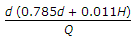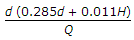# Civil Engineering - Waste Water Engineering - Discussion

### Discussion :: Waste Water Engineering - Section 1 (Q.No.21)

21.

The detention time of a circular tank of diameter d and water depth H, for receiving the sewage Q per hour, is

 [A].[B].[C].[D].Explanation:

No answer description available for this question.

 Dave said: (May 7, 2017) How? please explain the derivation.

 Bandlaravichandra said: (May 26, 2018) The Units of detention time is -sec.

 Subbu said: (Jul 30, 2018) The unit of detention time is sec. So we have 4 options can choose first one. (d^2=m^2 ) * ( (d=m)+(h=m))==m^3. m^3=volume. Q=m^3/sec.

 Naga Sai said: (Nov 1, 2018) Simple logic, thanks @Subbu.

 Sandeep Kumar said: (Jan 13, 2021) Thanks for explaining.

 Hima said: (Aug 11, 2021) Detention time (t) is equal to Volume of circular tank divided by rate of flow (Q).